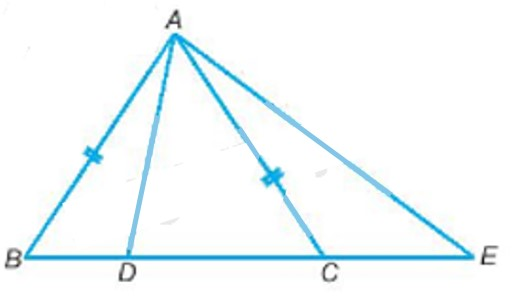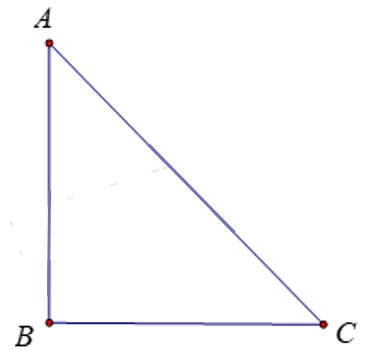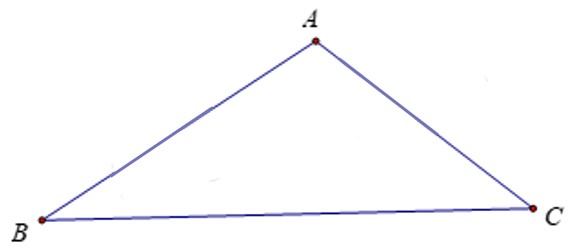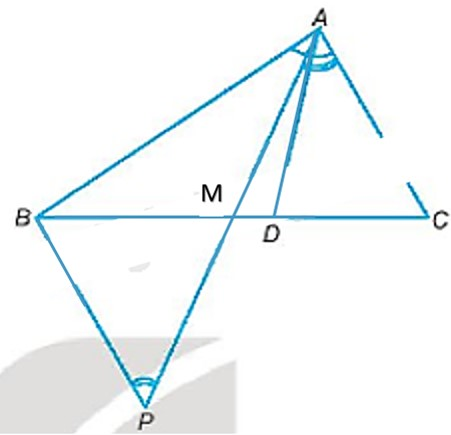## Solving SBT Lesson 31 Relationship between angle and opposite side in a triangle (Chapter 9 Math 7 Connection) – Math Book

### Solve problem 9.1 on page 48 Math 7 SBT Connecting knowledge volume 2 – KNTT

Triangle ABC has the longest side BC. Prove that the measure of angle A is greater than or equal to $${60^0}$$

Detailed explanation guide

Solution method:

Apply the relationship between sides and angles in triangles: The angle opposite the larger side is the larger angle.

Detailed explanation:

Since side BC is the longest, angle A is the largest (the relationship between the side and the angle in the triangle)

$$\Rightarrow$$$$\left\{ \begin{array}{l}\widehat A \ge \widehat B\\\widehat A \ge \widehat C\end{array} \right.$$

If $$\widehat A < {60^0} \Rightarrow \widehat B < {60^0};\widehat C < {60^0}$$

$$\Rightarrow \widehat A + \widehat B + \widehat C < {60^0} + {60^0} + {60^0} = {180^0}$$ (Nonsense)

So $$\widehat A \ge {60^0}$$

–>

— *****

### Solve lesson 9.2 on page 48 Math 7 SBT Connecting knowledge volume 2 – KNTT

Let ABC be an isosceles triangle at A, two points D and E lie on the line BC, D lies between B and C, C lies between D and E. Prove that AD < AC < AE.

Detailed explanation guide

Solution method:

– Consider AD perpendicular to BC

-AD is not perpendicular to BC

-Indicate obtuse angles

-Apply the relationship between sides and angles in triangles

Detailed explanation:TH1: $$AD \bot BC$$

Then: AC is the hypotenuse, AD is the side of the right angle

TH2: AD is not perpendicular to BC.

In two complementary angles ADB and ADC have an obtuse angle (Figure 9.12): triangle ADB is an obtuse triangle

Side AB is opposite obtuse angle ADB so AD < AB = AC (relationship between side and angle in triangle)

Conversely, the proof is similar when triangle ADC is an obtuse triangle: AD < AC

So we always have AD < AC (1)

Consider triangle ACE whose angle ACE is obtuse (compensate for acute angle ACB).

So AE > AC (the relationship between sides and angles in a triangle) (2)

From (1) and (2) deduce AD ​​< AC < AE.

–>

— *****

### Solve problem 9.3 page 48 SBT Math 7 Connecting knowledge volume 2 – KNTT

Explain why in a right triangle the hypotenuse is the longest and in an obtuse triangle the side opposite the obtuse angle is the longest side.

Detailed explanation guide

Solution method:

In a right triangle, the right angle is the largest angle because the other two angles are acute, so the side opposite it is the longest hypotenuse.

Similarly, in an obtuse triangle with one obtuse angle, the other two angles are acute, so the obtuse angle is the largest angle; So the side opposite the obtuse angle is the largest side.

Detailed explanation:

Consider triangle ABC, right angled at B:$$\widehat B = {90^0} \Rightarrow \left\{ \begin{array}{l}\widehat B > \widehat A\\\widehat B > \widehat C\end{array} \right.\ ) The side opposite the right angle is AC . So the hypotenuse AC is the largest (the relationship between the side and the angle in the triangle) – Consider an obtuse triangle ABC with angle A being an obtuse angle:\( \Rightarrow \widehat B,\widehat C$$ is an acute angle.

$$\Rightarrow \widehat A$$ is the largest angle.

The side opposite angle A is side BC .

$$\Rightarrow BC$$ is the largest side (the relationship between sides and angles in a triangle)

–>

— *****

### Solve problem 9.4 page 48 SBT Math 7 Connecting knowledge volume 2 – KNTT

Let ABC be a triangle with AB > AC. Let M be the midpoint of side BC.

a) Compare the two angles MAB and MAC.

b) The bisector of angle BAC intersects BC at D. Question D belongs to line segment MB or line segment MC? Why?

Detailed explanation guide

Solution method:

a)

– Take point P such that M is the midpoint of AP.

-Prove: $$\Delta AMC = \Delta PMB\left( {c – g – c} \right)$$

-Prove: $$\widehat {MPB} > \widehat {MAB}$$

Detailed explanation:a)

Take a point P such that M is the midpoint of AP.

Consider $$\Delta AMC$$ and $$\Delta PMB$$ have:

AM = PM

MC = MB

$$\widehat {AMC} = \widehat {PMB}$$

$$\Rightarrow \Delta AMC = \Delta PMB\left( {c – g – c} \right)$$
$$\Rightarrow \left\{ \begin{array}{l}AC = PB\\\widehat {MAC} = \widehat {MPB}\end{array} \right.$$

Since AB > AC then AB > PB

Consider triangle ABP with AB > PB

$$\begin{array}{l} \Rightarrow \widehat {MPB} > \widehat {MAB}\\ \Rightarrow \widehat {MAC} > \widehat {MAB}\end{array}$$

b)

We have: AD is the bisector of angle BAC so $$\widehat {DAB} = \widehat {DAC} = \dfrac{{\widehat {BAC}}}{2} \Rightarrow \widehat {BAC} = 2\ widehat {DAC}$$

Again there are:

$$\begin{array}{l}\widehat {MAC} > \widehat {MAB}\left( {cmt} \right)\\ \Rightarrow \widehat {MAC} + \widehat {MAC} > \widehat {MAB } + \widehat {MAC}\\ \Rightarrow 2\widehat {MAC} > \widehat {BAC}\\ \Rightarrow 2\widehat {MAC} > 2\widehat {DAC}\\ \Rightarrow \widehat {MAC} > \widehat {DAC}\end{array}$$

So D belongs to the line segment MC.

–>

— *****